American Journal of Theoretical and Applied Statistics
Volume 4, Issue 6, November 2015, Pages: 539-546

Modelling Oil Price Risk

Mwelu Susan, Anthony Gichuhi Waititu

School of Mathematical Sciences, Jomo Kenyatta University of Agriculture and Technology, Nairobi, Kenya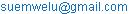(M. Susan)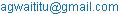(A. G. Waititu)

Mwelu Susan, Anthony Gichuhi Waititu. Modelling Oil Price Risk.American Journal of Theoretical and Applied Statistics.Vol.4, No. 6, 2015, pp. 539-546. doi: 10.11648/j.ajtas.20150406.25

Abstract: The energy sector is regarded as a key driving force for all other sectors in the economy. This can be attributed to oil being the global main source of energy as well as oil prices having a significant impact on financial markets and world economies. With the emergence of relatively free oil markets, prices are vulnerable to high shifts resulting in increased exposure to price risk. This research project focuses on the oil markets with two main oil price benchmarks being used: Brent blend of Europe and WTI of United States of America. As opposed to estimating a single distribution for the entire return series generating process this research project focuses on the tails of the distributions using limit laws from the Extreme Value Theory. A two stage GARCH-EVT approach is preferred in the study. The focus is on the peak over threshold method for analysing the generalized Pareto distributed exceedances over some significantly high threshold. The results of this study reveal that oil prices are highly volatile, heteroscedastic and fat-tailed. In addition the GPD fits the tails adequately well and is used to estimate associated tail risks at sufficiently high probabilities.

Keywords: Value-at-Risk, Oil Price Risk, GPD, Extreme Value Theory

Contents

1. Introduction

The oil market is arguably one of the largest commodity markets in the world. This can be attributed to oil being the main source of energy globally. Financial analysts believe that oil price fluctuations have considerable consequences on businesses, governments and the global economic activity.  argues that changes in oil prices have an impact on economic activity. Economies that depend on oil for industrial purposes experience significant uncertainty during periods of high volatility resulting in high volatility of exports and consequently government revenues. For instance, higher crude oil prices contribute to inflation and results to recession in oil-consuming countries.

The collapse of OPEC-administered pricing system in 1985 led to the rise of competition and de-regulation resulting in relatively free oil markets characterized by high degree of price volatility.  noted that since 1986 the trend in oil prices has significantly changed from what happened prior in that large price decreases are common. Oil price shifts could result from reasons such as OPEC policies, imbalances between physical supply and demand as well as war and political uncertainty in oil producing countries.  show the impact of oil-related events to oil price volatility. Their results indicate that the impact of the global financial crisis on oil price returns is significantly negative, while the impact of the Libyan war and hurricanes is significantly positive. However, the reactions of oil price returns to different OPEC production announcements are inconsistent.

Oil price risk is a major risk faced in the oil markets. This refers to the probability of loss occurring from adverse movements in the market price of crude oil. As a result, risk managers are faced with the challenge of implementation of effective risk models that have the capability to allow for rare events. The risk of extreme events is related to the tails of a distribution. Thus, a crucial challenge in getting good risk measure estimates is to be able to estimate the tail of the underlying distribution as accurately as possible. The value-at-risk (VaR) approach is used mainly for risk management as it measures the worst anticipated loss over a period for a given probability and under normal market conditions.

2. Related Works

Similarly to the stylized facts of financial time series, oil prices have been shown to possess unique properties.  show that oil prices exhibit excess kurtosis and that volatility clusters over time. In addition, like other financial time series, crude oil series are characterized by fat tail distribution, volatility clustering, asymmetry and mean reversion . The implication of excess kurtosis is that there is more mass in the tail areas than can be explained by the normal distribution.

 Quantified risk using VaR on Brent crude oil prices. They presented three kinds of VaR methods i.e. the historical simulation standard approach, the historical simulation with ARMA forecasts (HSAF) approach and the semi-parametric GARCH approach. They argue that although the historical simulation approach does not depend on any distribution of the underlying returns, the approach assumes that what happened in the past is a reflection of what will happen in the future. However, this may not necessarily be the case within the dynamic oil markets. Future risks may not necessarily be the same as historical risks more so in the case where high price shifts are observed. They further noted that the largest source of error in the parametric approaches used in their study was the assumption that returns are normally distributed.

EVT has been widely used in various fields among them including finance . It is important to note that EVT assumes the data under study are independently and identically distributed. A stylized fact of financial log-returns is that they tend to exhibit dependence in the second moment. In order to assess the risk of many financial transactions, estimates of asset return volatility are required.  suggest a two stage approach to address dependence of observations in applying extreme models to estimate tail risk.

First, fit a GARCH-type model to the returns data by quasi-maximum likelihood. The second stage of the approach is to apply EVT to the GARCH residuals. As opposed to EVT, GARCH models do not focus directly on the tail returns. Instead by acknowledging the tendency of volatility to be time dependent, GARCH models explicitly model conditional volatility as a function of past conditional volatilities and returns .

Asymmetric GARCH models are used to examine the probable existence of leverage effects. Leverage effect refers to negative and positive shocks having varied impacts on volatility i.e. negative correlation between volatility and returns. This is an important aspect that must be incorporated if present.  used the univariate GARCH models to measure uncertainty associated with Brent crude oil prices. The preferred model was a symmetric GARCH (1,3) as asymmetric leverage effects were not found.  compared the performance of both symmetric and asymmetric volatility models for oil price using daily returns of WTI. Their findings indicate that, based on the appropriate model selection criteria, the asymmetric. GARCH models appear superior to the symmetric ones in dealing with oil price volatility.

Financial disasters in the past have shown that indeed billions of dollars can be lost because of poor supervision and management of financial risks .

As opposed to other models that focus on the entire distribution, EVT focuses on the tail distribution of the returns. It provides a flexible and simple parametric model for capturing tail related behaviours. For that reason, it is not surprising that the extreme value based VaR is superior to the traditional variance-covariance and non-parametric methods in estimating extreme risks .

The link between EVT and risk management is that EVT methods fit extreme quantiles better than the conventional approaches for heavy-tailed data .

The main objective is to quantify the outcomes of a stochastic process which have a small probability of occurrence whose effects are severe. VaR describes the loss that can occur over a given period at a given confidence level, due to exposure to market risk.

3. Methodology

 suggested the ARCH model as an alternative to the standard time series treatments that cannot adequately explain the property of heteroscedasticity. The AR comes from the fact that these models are autoregressive models in squared returns. The conditional comes from the fact that in these models, next period’s volatility is conditional on information this period.

 extended the ARCH model of  to the generalized ARCH (GARCH) model. The virtue of this approach is that a GARCH model with a small number of terms appears to perform as well as or better than an ARCH model with many terms. In a GARCH model, returns are assumed to be generated by a stochastic process with time-varying volatility. This implies that the conditional distributions change over time in an auto-correlated way and the conditional variance is an autoregressive process. GARCH models provide an accurate assessment of variances and co-variances through their ability to model time-varying conditional variances as they assume that the conditional variance is a deterministic linear function of past squared innovations and past conditional variances. A GARCH (p,q) model takes the form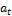=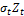, where: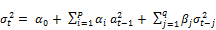(1)

Where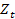, is a sequence of i.i.d random variables and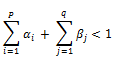to ensure stationarity of the volatility process.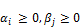The non-negativity restrictions on the parameters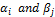ensure positivity of the variance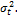The lag length of the GARCH model is selected by the use of the Akaike Information Criterion (AIC) which is a penalizing criterion.

The model with the lowest AIC value is selected to model the volatility process.

Modelling time series with leptokurtic characteristics and volatility clusters has been widely studied leading to extensions of the initial GARCH model proposed by . One particular characteristic of the standard GARCH model is that it assumes positive shocks are equal to negative shocks of the same magnitude thereby having the same effect on volatility. (The signs of innovations have no effect on conditional volatility as only the squared innovations enter the conditional variance equation.)

This aspect is referred to as the leverage effect.

In accounting for conditional heteroscedasticity in oil return series it is important to take account of any present leverage effects to avoid biased and misleading results . This study uses the asymmetric GJR-GARCH to check for leverage effect.

The volatility process of the GJR-GARCH model is given by: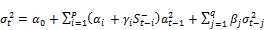(2)

Where,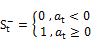The supposed effect of the returns on the conditional variance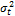is different according to the sign of the innovation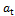. Hence the reason why the variable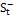is introduced to the model. This implies that the model accommodates the presence of leverage effect.

Diagnostic tests for the selected GARCH model is done through checking for serial correlation in the standardized residuals of the selected model.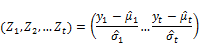These should be i.i.d.

Distribution of exceedances

The peaks over threshold method is demonstrated in figure 1. The method models the exceedances above a sufficiently high threshold u.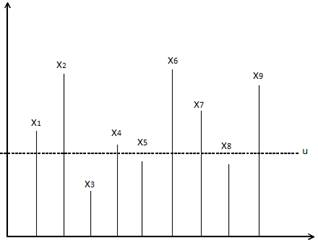Figure 1. Peaks over threshold method.

Let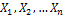be a sequence of i.i.d random variables from an unknown distribution function F. The interest is in estimating the distribution function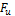of the excess values of X over a high threshold u. Let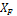be the finite or infinite right endpoint of the distribution F.

Then the conditional distribution function of the excesses over the threshold u can be defined by;(3)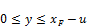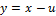are the excesses and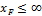is the right endpoint of F, that is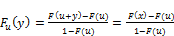(4)

Theorem: For a certain class of distributions, Balkema and De Haan (1974) and Pickands (1975) showed that the Generalized Pareto Distribution (GPD) is the limiting distribution for the distribution of excesses as the threshold tends to the right endpoint.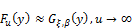The GPD is given by: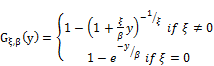(5)

For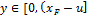if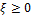and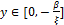if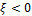However, the choice of a threshold is crucial. There is a challenge between setting a high threshold value that reduces the sample size to an insufficient level to meet the asymptotic properties or setting a low threshold level that ends up with a sizeable sample size but with more of the non-extreme values in the estimation. Various methods can be considered when selecting an appropriate threshold. One of the methods is the graphical representation. The empirical mean excess function which refers to the sum of excesses over threshold u divided by the number of data points exceeding the threshold is defined as: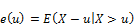A plot of the mean excess function is used where an appropriate threshold is selected at the point the plot tends to be linear and positively sloped.

Upon selection of an appropriate threshold the returns can then be modelled using the GPD with references to the following equations.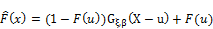(6)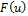can be approximated non-parametrically by: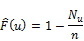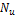represents the number of exceedances above the threshold u and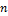represents the sample size.

Solving for the distribution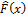we obtain: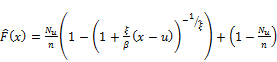(7)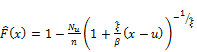(8)

Inverting equation 8 for a given probability p we have the value at risk given by: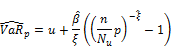This study focuses on estimating price risk with a focus on the oil market. Extreme Value modelling provides a statistically justifiable model to capture the tails of the underlying data generating process.

The volatility model is combined with EVT to estimate conditional extreme quantile over a one day period. The expected shortfall is a measure used to quantify the worst loss given that the value at risk was exceeded.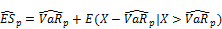4. Empirical Results

The data used in the study consists of daily prices of two main benchmarks (WTI and BRENT Blend) for the period 1986-2015.

4.1. Data Exploration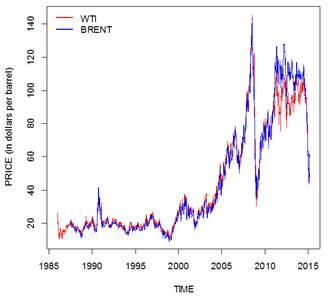Figure 2. Trends in crude oil prices.

Figure 2 shows the general trend in oil prices. Crude oil prices for both series are fairly stable between 1986-1999. From 1999 up until mid-2008, the price significantly rises to a peak of \$145 per barrel. However, a major shock is observed between 2007 and 2008 where the prices are consistently on a downward trend. Lows of \$30.28 and \$30.41 are observed for WTI and Brent respectively. Coincidentally this is about the same period as the global financial crisis of 2007-2010. The prices then rebound to an upward trend with significant volatility being experienced. Up until mid- 2014 the prices range between \$90-\$120.

Towards the end of 2014 into early 2015, there is a decline in prices to a low of between \$46.09-\$44.08 per barrel. This can be attributed to increased production of American shale oil that created a surplus in the market resulting to decline in prices.

Crude oil prices were transformed into log returns. Unlike the raw price data log returns tend to evolve as a stationary process. At 5% level of significance both return series are found to be stationary.

Return series for both data sets are as shown in figure 3.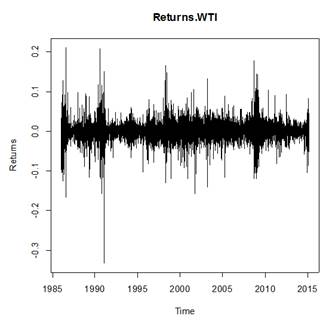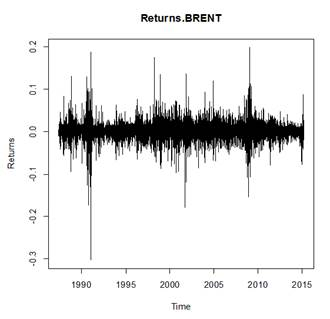Figure 3. WTI and Brent return series.

Both return series exhibit a kurtosis greater than that of a normal distribution and negative skewness. The implication of excess kurtosis is that there is more mass in the tail area than can be explained by the normal distribution. Similarly negative skewness indicates that the left tails tend to be heavier than the right tails.

Table 1. Descriptive statistics of returns.

 WTI Brent Data length 7456 7110 Mean 0.000606 0.0001864 Std.dev 0.024784 0.022443 Skewness -0.2318976 -0.489066 Kurtosis 10.61066 11.14741 Jarque-Bera 35044 36840 ADF (Statistic) -18.287 -17.919 ADF (p-value) 0.01 0.01

The Akaike’s Information criterion (AIC) was used to select the best model based on the lowest value obtained. The GARCH (1, 1) model was selected as presence of leverage effects was not detected through the asymmetric GJR-GARCH (1, 1) model.

The estimated GARCH(1,1) model parameters are presented in table 2.

Table 2. GARCH (1, 1) model parameters.

 α0 α1 β1 WTI 0.000006 0.095731 0.899545 BRENT 0.000003 0.072379 0.924442

At 5% level of significance all parameters were found to be significant. The standard GARCH(1,1) model yielded the smallest AIC indicating that it is adequate in describing the volatility process of both return series. The estimated leverage effect in the GJR-GARCH model was found to be positive and insignificant at 5% level of significance.

The GARCH(1,1) model is thus found to be adequate in describing the volatility process.

Correlograms of the standardized residuals and squared standardized residuals revealed absence of serial correlation. The standardized residuals are found to be i.i.d.

4.2. Extreme Value Theory

To capture for the assymetry in the tails, we analyse the tails separately as the left and right tail as per . Appropriate threshold levels are selected using the sample mean excess plots.The values from which the plots tend to linearity and a positive slope are selected. The selected thresholds, number of exceedances and estimated generalized pareto model parameters are presented in tables 3 and 4.

Figure 5 and 6 shows the fit of the excedandances to the fitted GPD model for the right and left tails respectively for both data series. The plots exhibit a generally good fit to the exceedances on the estimated model for the tails in both data series. The points on the qq plot lay on the straight line representing the estimated model indicating a good fit.

Point estimates of the risk measures are then estimated at significantly high probability levels.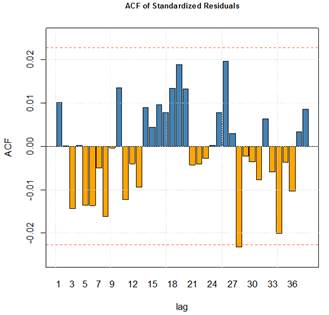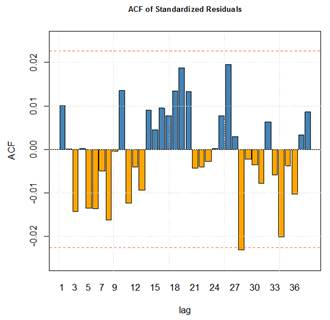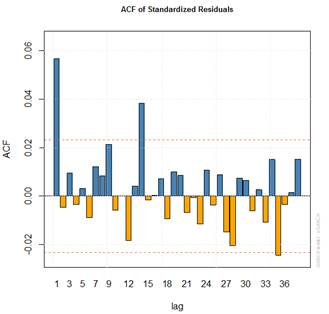Note: WTI is presented on the top panel, Brent on the bottom panel

Figure 4. Correlograms for standardized residuals and squared standardized residuals.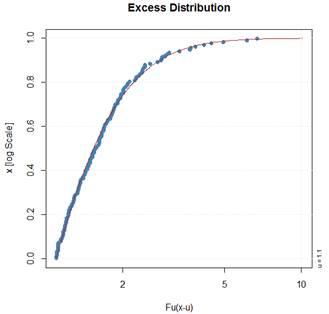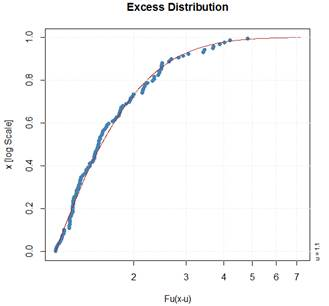Figure 5. Excess distribution for WTI left and right tails respectively.

Table 3. Estimated GPD parameters for Brent right and left tails.

 Threshold No.of exceedances ξ β Right tail 1.3 60 -0.2409189 1.0862891 Left tail 1.5 39 0.03818405 0.59389695

Table 4. Estimated GPD parameters for WTI right and left tails.

 Threshold No.of exceedances ξ β Right tail 1.1 111 0.07711093 0.65462851 Left tail 1.1 144 0.1839816 0.5911181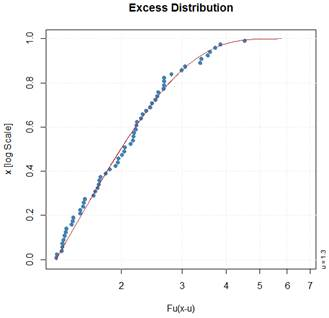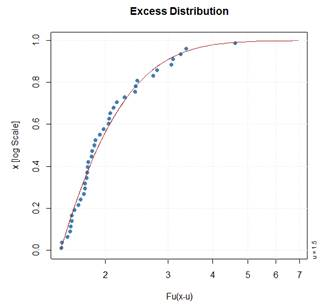Figure 6. Excess distribution for Brent right and left tails respectively.

Table 5. Estimated risk measures for WTI left and right tails.

 Probability(p)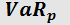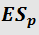Left tail 0.990 3.254303 4.464347 0.995 3.984274 5.358920 0.999 6.085371 7.933794 Right tail 0.990 2.973787 3.839869 0.995 3.542873 4.456534 0.999 4.987753 6.022216

Table 6. Estimated risk measures for Brent left and right tails.

 Probability(p)Left tail 0.990 3.254303 4.464347 0.995 3.984274 5.358920 0.999 6.085371 7.933794 Right tail 0.990 2.973787 3.839869 0.995 3.542873 4.456534 0.999 4.987753 6.022216

The results in Table 5 indicate that, with a confidence band of 99.0% the VaR values for the WTI left and right tail are 3.254303 and 2.973787 respectively. Say with a financial position of \$1 million dollars the value at risk values are \$32,543 and \$29,737 respectively. Given that these values are exceeded then, the expected shortfall values are 4.464347 and 3.839869. For the said financial position then the expected shortfall values are \$44, 6434 and \$38,398 respectively for the left and right tails.

Similarly, with a confidence band of 95.0% the VaR values for the WTI left and right tails are 3.984274 and 3.542873 respectively. With a financial position of \$1 million dollars the value at risk values are \$39,842 and \$35,428 respectively. Given that these values are exceeded then, the expected shortfall values are 5.35892 and 4.456534. For the said financial position then the expected shortfall values are \$53,589 and \$44,565 respectively for the left and right tails.

For higher confidence intervals such as 99.9% the Value at risk is 6.085371 and given that this is exceeded the expected shortfall is 7.933794.

The results in Table 6 indicate that, with a confidence band of 99.0% given a financial position of \$1 million dollars the value at risk is \$28,241 and \$32,387 for the Brent left and right tails respectively. Similarly, with a higher confidence band of 99.5 the VaR is \$32,767 and \$36,340 for the left and right tails respectively. Similar inferences can be drawn for the other values.

5. Discussion

This study aimed at modelling oil price risk. The GARCH-EVT model was used to achieve the main study objective. The results revealed key characteristics of crude oil prices within the study period. The return series are mean reverting, leptokurtic, negatively skewed and fat-tailed relative to the Gaussian distribution. The standard GARCH and asymmetric GJR-GARCH models were used to model the volatility process. However, the asymmetric effect was found to be insignificant in both time series, hence the standard GARCH (1,1) concluded to be adequate in characterising the volatility process as per the Akaike's selection criteria. As opposed to estimating a single distribution for the entire series, the tails were modelled following limit laws from extreme value theory. Due to asymmetry in the tails, analysis of both left and right tails of the standardized residuals of the GARCH (1, 1) model exceeding respective thresholds showed that excesses are i.i.d and can be modelled by the GPD. The estimated shape parameters further indicated presence of heavy tails except for the Brent right tail that yielded -0.2409189 for the shape parameter indicating a light tail. However, the left tails appear to be heavier as compared to the right tails as was observed in the shape index of the GPD. This is an indication that extreme events are mainly within the left tail as compared to the right tail. Diagnostic plots for the estimated GPD parameters indicated that the models fit adequately well. Point estimates of extreme tail risk measures were then estimated at sufficiently high probability levels.

6. Conclusion and Recommendation

Presence of high volatility within oil markets leads to the requirement of implementation of effective risk models.  results on tail distribution modelling show that tail- related risk measures such as Value-at-Risk and expected shortfall can be modelled using extreme value theory. A conditional approach is favoured in this study as temporal dependence within crude oil daily returns was detected in the data series. EVT provides sound statistical methodology for modelling tail related risk measures. Application of EVT captures the heavy-tailed behaviour of the crude oil returns and the asymmetric characteristics in the distribution is captured by treating the left and right tails separately.

This study favours the peaks over threshold method as it tends to utilize data more efficiently. A major challenge in this method is the selection of an appropriate threshold. This study adopts the mean excess function to select appropriate thresholds for the respective tails.

By assessing empirical excess distribution functions with associated theoretical distributions, we find the goodness of fit in tail modelling. Extreme tail risk measures are estimated at significantly high confidence levels such as 99.5%, 99% and 99.9%.

The EVT-based Value-at-Risk approach adopted in this study provides quantitative information for analysing the extent of potential extreme risks in oil markets, particularly based on two crude oil markets. Generally the WTI benchmark yields higher risk measures than the Brent. This leads to the conclusion that the WTI faces an increased exposure to price risk as compared to the Brent.

For an oil market investor, country or firm, the risk measure values would be a great source of information for hedging purposes, planning purposes and taxation regulations by governments.

Due to the dynamic nature of the oil markets, time varying shape and scale parameters can be considered for further studies. In addition, further methods of threshold selection can be considered.

Abbreviations

OPEC: Organization of Petroleum Exporting Countries

POT: Peaks over Threshold

VaR: Value at Risk

ES: Expected Shortfall

ARCH: Autoregressive Conditional Heteroscedasticity

GARCH: Generalized Autoregressive Conditional Heteroscedasticity

i.i.d: Independent and identically distributed

EVT: Extreme Value Theory

WTI: West Texas Intermediate

References

1. Aragonés, J., Dowd, K., and Blanco, C. (2000). Extreme value var. Derivatives Week, pages 7–8.
2. Bollerslev, T. (1986). Generalized autoregressive conditional heteroscedasticity. Journal of econometrics, 31(3):307–327.
3. Cabedo, J. D. and Moya, I. (2003). Estimating oil price value at risk using the historical simulation approach. Energy Economics, 25(3):239–253.
4. Embrechts, P., Klüppelberg, C., and Mikosch, T. (1997). Modelling extremal events, volume 33. Springer Science & Business Media.
5. Engle, R. F. (1982). Autoregressive conditional heteroscedasticity with estimates of the variance of united kingdom inflation. Econometrica: Journal of the Econometric Society, pages 987– 1007.
6. Gencay, R. and Selcuk, F. (2004). Extreme value theory and value-at-risk: Relative performance in emerging markets. International Journal of Forecasting, 20(2):287–303.
7. Giot, P. and Laurent, S. (2003). Market risk in commodity markets: a var approach. Energy Economics, 25(5):435–457.
8. Ji, Q., & Guo, J. F. (2015). Oil price volatility and oil-related events: An Internet concern study perspective. Applied Energy, 137, 256-264.
9. Këllezi, E. and Gilli, M. (2000). Extreme value theory for tail-related risk measures. FAME.
10. Krehbiel, T. and Adkins, L. C. (2005). Price risk in the nymex energy complex: an extreme value approach. Journal of Futures Markets, 25(4):309–337.
11. Kuper, G. H. (2002). Measuring oil price volatility. Available at SSRN 316480.
12. Marimoutou, V., Raggad, B., and Trabelsi, A. (2009). Extreme value theory and value at risk: application to oil market. Energy Economics, 31(4):519–530.
13. McNeil, A. J. and Frey, R. (2000). Estimation of tail-related risk measures for heteroscedastic financial time series: an extreme value approach. Journal of empirical finance, 7(3):271–300.
14. Sadorsky, P. (1999). Oil price shocks and stock market activity. Energy Economics, 21(5):449– 469.
15. Salisu, A. A. and Fasanya, I. O. (2012). Comparative performance of volatility models for oil price. International Journal of Energy Economics and Policy, 2(3):167–183.
16. Sauter, R. and Awerbuch, S. (2003). Oil price volatility and economic activity: a survey and literature review. IEA Research Paper.

 Contents 1. 2. 3. 4. 4.1. 4.2. 5. 6.
Article ToolsAbstractPDF(531K)